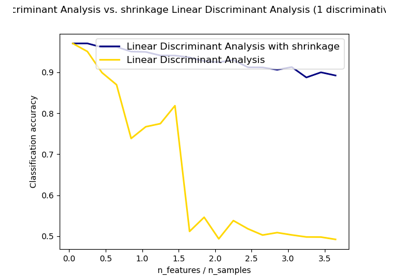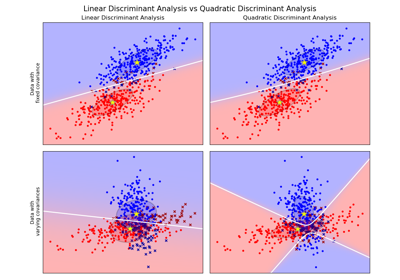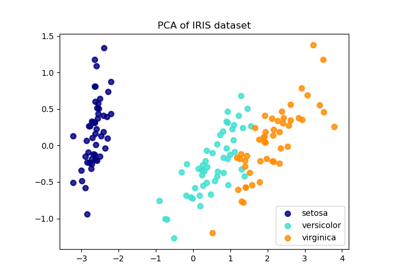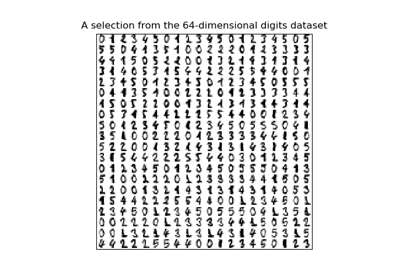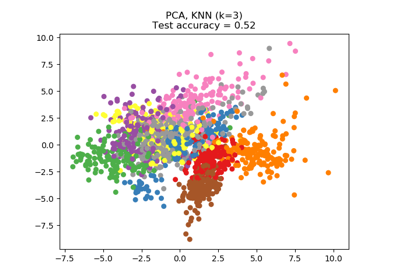# sklearn.discriminant_analysis.LinearDiscriminantAnalysis¶

class sklearn.discriminant_analysis.LinearDiscriminantAnalysis(solver='svd', shrinkage=None, priors=None, n_components=None, store_covariance=False, tol=0.0001)[source]

Linear Discriminant Analysis

A classifier with a linear decision boundary, generated by fitting class conditional densities to the data and using Bayes’ rule.

The model fits a Gaussian density to each class, assuming that all classes share the same covariance matrix.

The fitted model can also be used to reduce the dimensionality of the input by projecting it to the most discriminative directions.

New in version 0.17: LinearDiscriminantAnalysis.

Read more in the User Guide.

Parameters
solverstring, optional
Solver to use, possible values:
• ‘svd’: Singular value decomposition (default). Does not compute the covariance matrix, therefore this solver is recommended for data with a large number of features.

• ‘lsqr’: Least squares solution, can be combined with shrinkage.

• ‘eigen’: Eigenvalue decomposition, can be combined with shrinkage.

shrinkagestring or float, optional
Shrinkage parameter, possible values:
• None: no shrinkage (default).

• ‘auto’: automatic shrinkage using the Ledoit-Wolf lemma.

• float between 0 and 1: fixed shrinkage parameter.

Note that shrinkage works only with ‘lsqr’ and ‘eigen’ solvers.

priorsarray, optional, shape (n_classes,)

Class priors.

n_componentsint, optional (default=None)

Number of components (<= min(n_classes - 1, n_features)) for dimensionality reduction. If None, will be set to min(n_classes - 1, n_features).

store_covariancebool, optional

Additionally compute class covariance matrix (default False), used only in ‘svd’ solver.

New in version 0.17.

tolfloat, optional, (default 1.0e-4)

Threshold used for rank estimation in SVD solver.

New in version 0.17.

Attributes
coef_array, shape (n_features,) or (n_classes, n_features)

Weight vector(s).

intercept_array, shape (n_classes,)

Intercept term.

covariance_array-like, shape (n_features, n_features)

Covariance matrix (shared by all classes).

explained_variance_ratio_array, shape (n_components,)

Percentage of variance explained by each of the selected components. If n_components is not set then all components are stored and the sum of explained variances is equal to 1.0. Only available when eigen or svd solver is used.

means_array-like, shape (n_classes, n_features)

Class means.

priors_array-like, shape (n_classes,)

Class priors (sum to 1).

scalings_array-like, shape (rank, n_classes - 1)

Scaling of the features in the space spanned by the class centroids.

xbar_array-like, shape (n_features,)

Overall mean.

classes_array-like, shape (n_classes,)

Unique class labels.

Notes

The default solver is ‘svd’. It can perform both classification and transform, and it does not rely on the calculation of the covariance matrix. This can be an advantage in situations where the number of features is large. However, the ‘svd’ solver cannot be used with shrinkage.

The ‘lsqr’ solver is an efficient algorithm that only works for classification. It supports shrinkage.

The ‘eigen’ solver is based on the optimization of the between class scatter to within class scatter ratio. It can be used for both classification and transform, and it supports shrinkage. However, the ‘eigen’ solver needs to compute the covariance matrix, so it might not be suitable for situations with a high number of features.

Examples

>>> import numpy as np
>>> from sklearn.discriminant_analysis import LinearDiscriminantAnalysis
>>> X = np.array([[-1, -1], [-2, -1], [-3, -2], [1, 1], [2, 1], [3, 2]])
>>> y = np.array([1, 1, 1, 2, 2, 2])
>>> clf = LinearDiscriminantAnalysis()
>>> clf.fit(X, y)
LinearDiscriminantAnalysis()
>>> print(clf.predict([[-0.8, -1]]))



Methods

 decision_function(self, X) Predict confidence scores for samples. fit(self, X, y) Fit LinearDiscriminantAnalysis model according to the given fit_transform(self, X[, y]) Fit to data, then transform it. get_params(self[, deep]) Get parameters for this estimator. predict(self, X) Predict class labels for samples in X. predict_log_proba(self, X) Estimate log probability. predict_proba(self, X) Estimate probability. score(self, X, y[, sample_weight]) Return the mean accuracy on the given test data and labels. set_params(self, \*\*params) Set the parameters of this estimator. transform(self, X) Project data to maximize class separation.
__init__(self, solver='svd', shrinkage=None, priors=None, n_components=None, store_covariance=False, tol=0.0001)[source]

Initialize self. See help(type(self)) for accurate signature.

decision_function(self, X)[source]

Predict confidence scores for samples.

The confidence score for a sample is the signed distance of that sample to the hyperplane.

Parameters
Xarray_like or sparse matrix, shape (n_samples, n_features)

Samples.

Returns
array, shape=(n_samples,) if n_classes == 2 else (n_samples, n_classes)

Confidence scores per (sample, class) combination. In the binary case, confidence score for self.classes_ where >0 means this class would be predicted.

fit(self, X, y)[source]
Fit LinearDiscriminantAnalysis model according to the given

training data and parameters.

Changed in version 0.19: store_covariance has been moved to main constructor.

Changed in version 0.19: tol has been moved to main constructor.

Parameters
Xarray-like, shape (n_samples, n_features)

Training data.

yarray, shape (n_samples,)

Target values.

fit_transform(self, X, y=None, **fit_params)[source]

Fit to data, then transform it.

Fits transformer to X and y with optional parameters fit_params and returns a transformed version of X.

Parameters
Xnumpy array of shape [n_samples, n_features]

Training set.

ynumpy array of shape [n_samples]

Target values.

**fit_paramsdict

Returns
X_newnumpy array of shape [n_samples, n_features_new]

Transformed array.

get_params(self, deep=True)[source]

Get parameters for this estimator.

Parameters
deepbool, default=True

If True, will return the parameters for this estimator and contained subobjects that are estimators.

Returns
paramsmapping of string to any

Parameter names mapped to their values.

predict(self, X)[source]

Predict class labels for samples in X.

Parameters
Xarray_like or sparse matrix, shape (n_samples, n_features)

Samples.

Returns
Carray, shape [n_samples]

Predicted class label per sample.

predict_log_proba(self, X)[source]

Estimate log probability.

Parameters
Xarray-like, shape (n_samples, n_features)

Input data.

Returns
Carray, shape (n_samples, n_classes)

Estimated log probabilities.

predict_proba(self, X)[source]

Estimate probability.

Parameters
Xarray-like, shape (n_samples, n_features)

Input data.

Returns
Carray, shape (n_samples, n_classes)

Estimated probabilities.

score(self, X, y, sample_weight=None)[source]

Return the mean accuracy on the given test data and labels.

In multi-label classification, this is the subset accuracy which is a harsh metric since you require for each sample that each label set be correctly predicted.

Parameters
Xarray-like of shape (n_samples, n_features)

Test samples.

yarray-like of shape (n_samples,) or (n_samples, n_outputs)

True labels for X.

sample_weightarray-like of shape (n_samples,), default=None

Sample weights.

Returns
scorefloat

Mean accuracy of self.predict(X) wrt. y.

set_params(self, **params)[source]

Set the parameters of this estimator.

The method works on simple estimators as well as on nested objects (such as pipelines). The latter have parameters of the form <component>__<parameter> so that it’s possible to update each component of a nested object.

Parameters
**paramsdict

Estimator parameters.

Returns
selfobject

Estimator instance.

transform(self, X)[source]

Project data to maximize class separation.

Parameters
Xarray-like, shape (n_samples, n_features)

Input data.

Returns
X_newarray, shape (n_samples, n_components)

Transformed data.

## Examples using sklearn.discriminant_analysis.LinearDiscriminantAnalysis¶